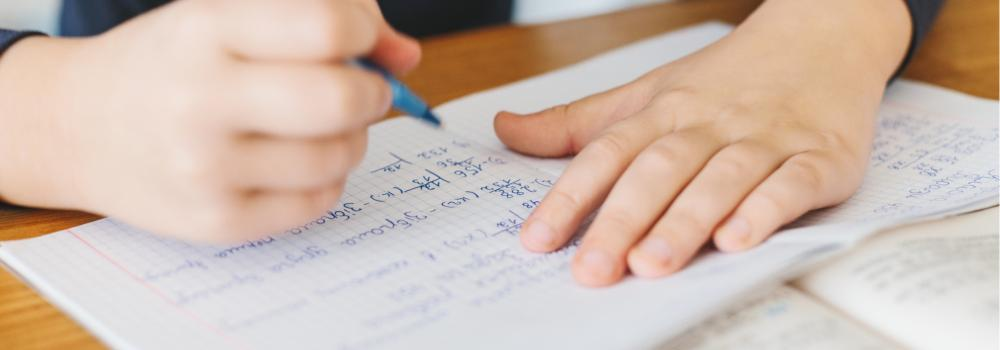# Secondary/7-9: Skills for Constant PracticeEach rung on the ladder has activities that may be copied from the level below or may be new activities, which allow you to support your child to keep important skills up to scratch.

### Bronze

• Multiplying and Dividing by 10 and 100
• Ordering Numbers
• Reading and Writing Large Numbers
• Linking Fractions. Decimals and Percentages
• Recording Multiplying and Dividing
• Probability Scale
• Symmetry
• Rotational Symmetry
• Line Graphs
• Pictograms

### Silver

• Special Types of Number
• Prime Factors
• Highest Common Factor
• Lowest Common Multiple
• Any Percentage of Any Number
• The Order of Calculations
• Addition and Subtraction of Fractions
• Rounding Decimals
• Negative Integers
• Cubed Numbers and Cube Roots
• Multiplication (Grid Method)
• Formal Recording Multiplication
• Formal recording Division

### Gold

• Powers
• Calculating with Standard Form
• Multiplying and Dividing by 10 and 100
• Reading and Writing Large Numbers
• Rounding Decimals
• Budgeting with Money
• Reflective Symmetry
• Rotational Symmetry
• Formal Recording Multiplication
• Formal recording Division

### Areas of study

This site uses cookies. You can opt out of receiving optional cookies at any time.